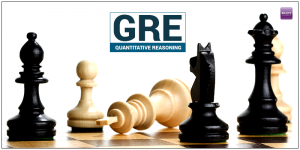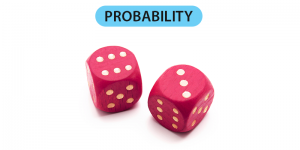# GRE Quantitative#### All About GRE Quantitative Reasoning

The Quantitative section tests the candidate’s competency in Maths. The questions involved are taken from topics like – Algebra, Geometry, …

#### GRE Quantitative Algebra – Solving Linear Equations

A system of equations is called as a set of linear equations, if it includes two or more linear equations …#### GRE Quantitative Data Analysis – Probability

Usually, you won’t be facing many probability questions in the GRE exam, and this is why many test aspirants start …

#### GRE Quantitative Arithmetic – Exponents & Roots

Exponents and roots are the basic topics that GRE tests from your grade school knowledge. So, one thing which is …

#### GRE Quantitative Algebra – Solving Linear Inequalities

Solving a question that contains only inequality, or only an equation that is specifically based on equations is rather a …

#### GRE Quantitative Arithmetic – Fractions

The practice of working with fractions that too without using a calculator was long forgotten since grade school. GRE demands …

#### GRE Quantitative Algebra – Applications

Algebra and its applications are quite vast, the boundaries of algebra are not confined to the simple problems of addition, …

#### GRE Quantitative: Geometry – Quadrilaterals

GRE gives special attention to the section of Quadrilaterals in the Quantitative reasoning section. It is important for every GRE …

#### GRE Quantitative: Geometry – Polygons

Polygons always give a shiver to most students but you don’t need to be afraid of it. Polygon is no …

#### GRE Quantitative: Algebra – Coordinate Geometry

Coordinate Geometry is the third most liked topic in the Quantitative Reasoning section of GRE. Usually the questions asked in …